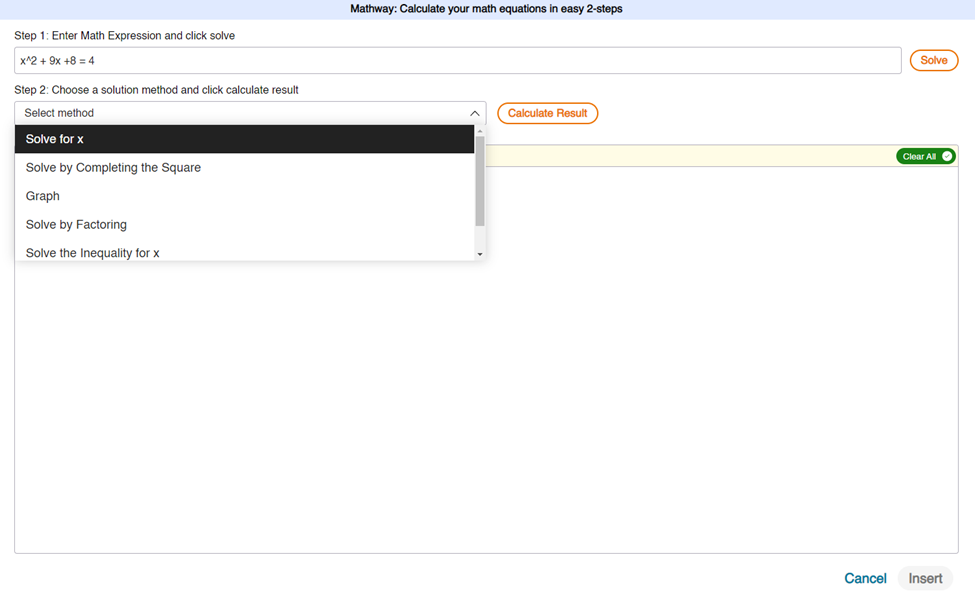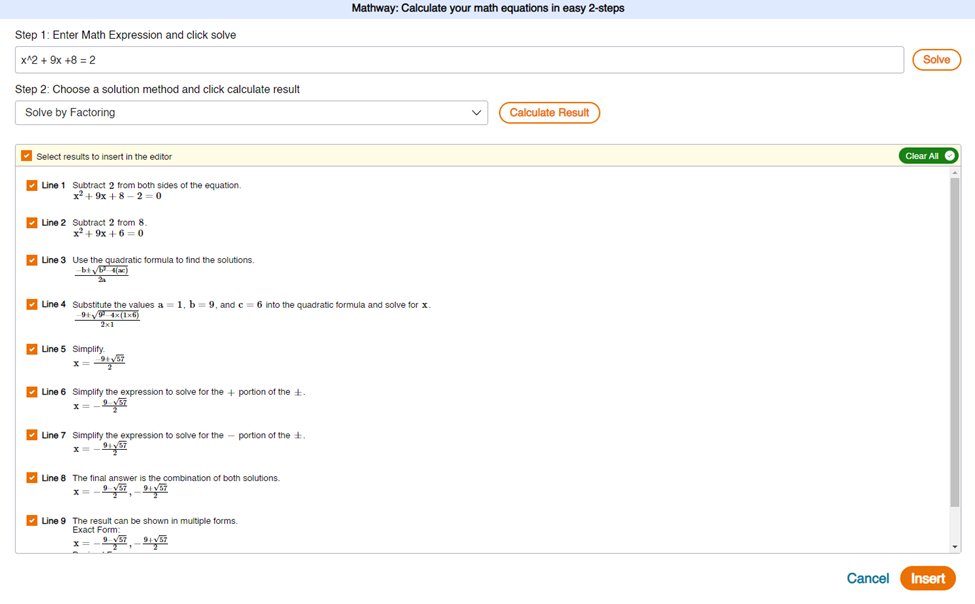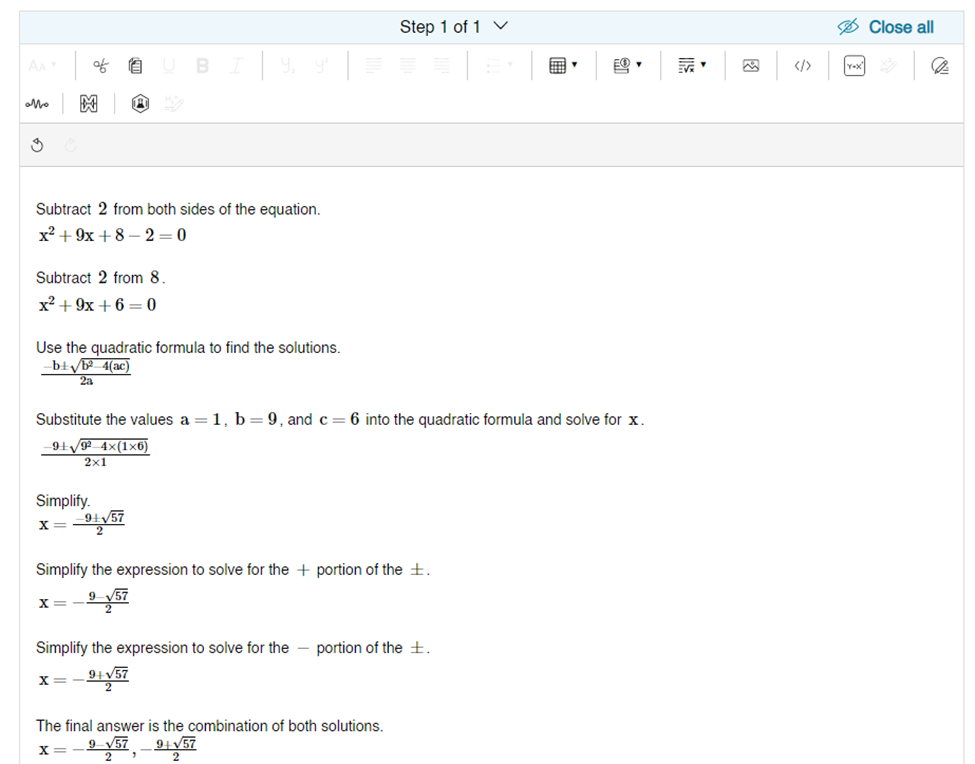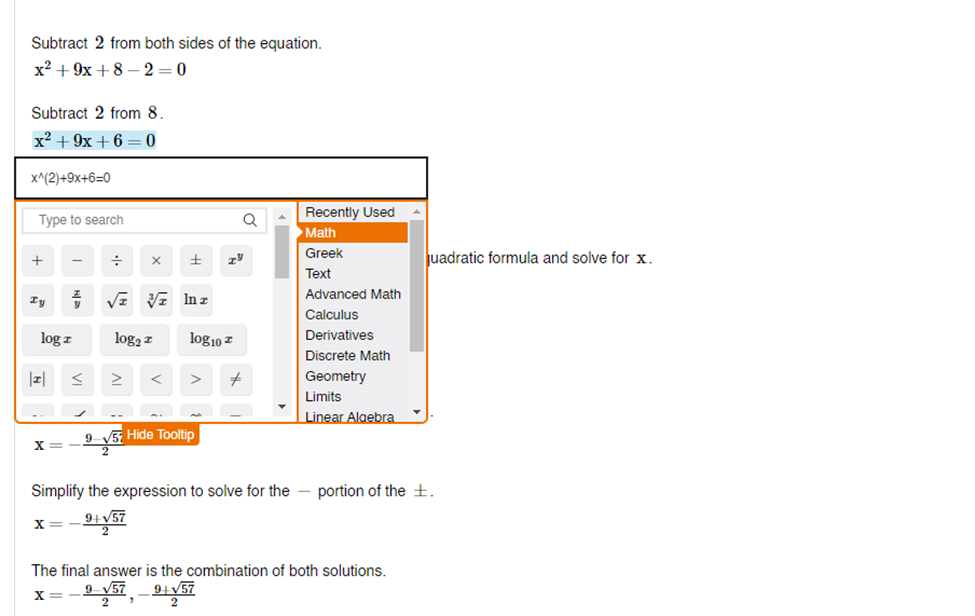# New Mathway Tool on Expert Platform 2.0: Solve Math Problems with Ease

Mathway Tool

Mathway tool automatically solves mathematical expressions and provides a step-by-step solution.

Key features:

Convenient toolbar access – Experts can access Mathway directly in EP 2.0 editor via a toolbar button.

ASCII math notation – Mathway tool accepts simple, standard keyboard mathematical expressions.

Quick insertion of solutions – Experts can insert the entire Mathway solution into the editor with a single button click.

Fully integrated with EP 2.0 Math tools – all math created by the Mathway tool is compatible with EP 2.0 Inline Math tool and can be modified by Experts.Figure 1 - Mathway modal showing multiple solution methods for solving a quadratic equationFigure 2 - Mathway modal populated with full solutionFigure 3 - Mathway solution inserted into the editorFigure 4 - Mathway expressions fully compatible and editable via EP 2.0 Inline Math tool